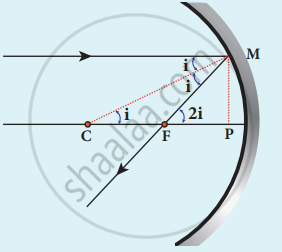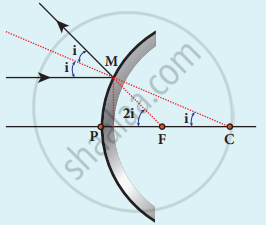Tamil Nadu Board of Secondary EducationHSC Science Class 12th

# Derive the relation between f and R for a spherical mirror. - Physics

Derive the relation between f and R for a spherical mirror.

#### Solution

1. C be the center of curvature of the mirror
2. F be the principal focus.
3. Line CM is normal to the mirror at M.
4. i be the angle of incidence
∠MCP = i and ∠MFP = 2 i(a) Concave mirror(b) Convex Mirror

ΔMCP,

tan i = "PM"/"PC"

ΔMFB, tan 2i = "PM"/"PF"

i = "PM"/"PC" and "2i" = "PM"/"PF"

2"PM"/"PC" = "PM"/"PF"; 2PF = PC

∵ PF = f

PC = R

2f = R (or) f = "R"/2

Concept: Spherical Mirrors
Is there an error in this question or solution?

Share